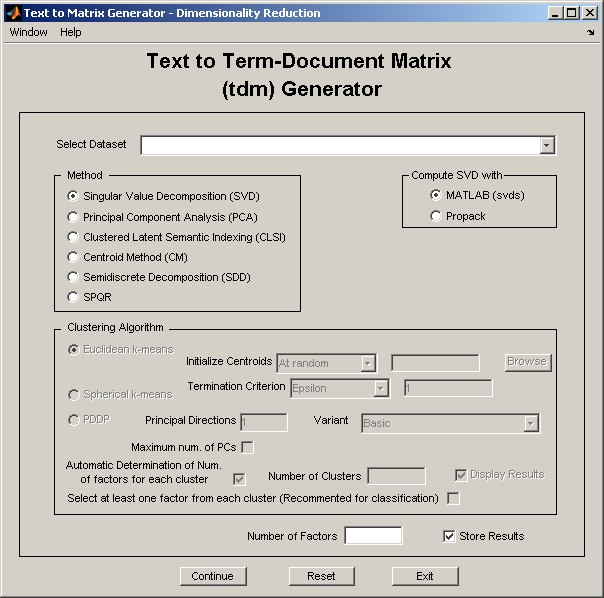## Dimensionality Reduction Module (tmg_gui)dr_gui is a graphical user interface for "Text to Matrix Generator" that can be used for applying a set of dimensionality reduction techniques to term-document matrices (TDM's) constructed from tmg_gui.

See a demonstration of dr_gui.

For complete up-to-date documentation visit the TMG Wiki:

 Field Name Default Description Select Dataset - Select the dataset. Singular Value Decomposition (SVD) ● Apply the SVD method. Principal Component Analysis (PCA) - Apply the PCA method. Clustered Latent Semantic Indexing (CLSI) - Apply the CLSI method. Centroid Method (CM) - Apply the CM method. Semidiscrete Decomposition (SDD) - Apply the SDD method. SPQR - Apply the SPQR method. MATLAB (svds) ● Check to use MATLAB function svds for the computation of the SVD or PCA. Propack - Check to use PROPACK package for the computation of the SVD or PCA. Euclidean k-means ● Check to use the euclidean k-means clustering algorithm in the course of CLSI or CM. Spherical k-means - Check to use the spherical k-means clustering algorithm in the course of CLSI or CM. PDDP - Check to use the PDDP clustering algorithm in the course of CLSI or CM. Initialize Centroids At random Defines the method used for the initialization of the centroid vector in the course of k-means. Possibilities are: initialize at random and supplly a variable of '.mat' file with the centroids matrix. Termination Criterion Epsilon (1) Defines the termination criterion used in the course of k-means. Possibilities are: use an epsilon value (default 1) and stop iteration when the objective function improvement does not exceed epsilon or perform a specific number of iterations (default 10). Principal Directions 1 Number of principal directions used in PDDP. Maximum num. of PCs - Check if the PDDP(max-l) variant is to be applied. Variant Basic A set of PDDP variants. Possibe values: 'Basic', 'Split with k-means', 'Optimat Split', 'Optimal Split with k-means', 'Optimal Split on Projection'. Automatic Determination of Num. of factors for each cluster ● Check to apply a heuristic for the determination of the number of factors computed from each cluster in the course of the CLSI algorithm. Number of Clusters - Number of clusters computed in the course of the CLSI algorithm. Display Results ● Display results or not to the command windows. Select at least one factor from each cluster - Use this option in case low-rank data are to be used in the course of classification. Number of factors - Rank of approximation. Store Results ● Check to store results. Continue - Apply the selected operation. Reset - Reset window to default values. Exit - Exit window.